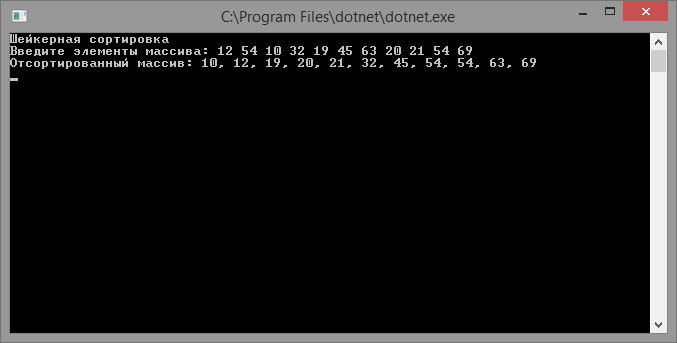# Shaker sort

Updated: 15.09.2019

Shuffle sorting (cocktail sort) or shaker sorting is an advanced type of bubble sorting in which the sorting is performed in two directions, changing direction with each pass.

## Description of the shaker sorting algorithm

Having analyzed the bubble sorting algorithm, you can notice:

• if there were no element exchanges while traversing part of the array, this part can be excluded, since it has already been sorted;
• when passing from the end of the array to the beginning, the minimum value is shifted to the first position, while the maximum element moves only one index to the right.

Based on the above ideas, we modify the sorting by the bubble as follows:

• at each iteration, we fix the boundaries of the part of the array in which the exchange takes place;
• the array is dispensed alternately from the beginning of the array to the end and from end to beginning;

In this case, the minimum element moves to the beginning of the array, and the maximum - to the end, after which the working area of ​​the array decreases.

## Shaker Sort Implementation

``````using System;

class program
{
// elements exchange method
static void Swap(ref int e1, ref int e2)
{
var temp = e1;
e1 = e2;
e2 = temp;
}

// sort by shuffle
static int[] ShakerSort(int[] array)
{
for (var i = 0; i < array.Length / 2; i++)
{
var swapFlag = false;
// pass from left to right
for (var j = i; j < array.Length - i - 1; j++)
{
if (array[j] > array[j + 1])
{
Swap(ref array[j], ref array[j + 1]);
swapFlag = true;
}
}

// pass from right to left
for (var j = array.Length - 2 - i; j > i; j--)
{
if (array[j - 1] > array[j])
{
Swap(ref array[j - 1], ref array[j]);
swapFlag = true;
}
}

// if there were no exchanges, exit
if (!swapFlag)
{
break;
}
}

return array;
}

static void Main(string[] args)
{
Console.WriteLine("Shaker Sort");
Console.Write("Enter elements of the array:");
var parts = Console.ReadLine().Split(new[] { "", ",", ";" }, StringSplitOptions.RemoveEmptyEntries);
var array = new int[parts.Length];
for (int i = 0; i < parts.Length; i++)
{
array[i] = Convert.ToInt32(parts[i]);
}

Console.WriteLine("Sorted array: {0}", string.Join(",", ShakerSort(array)));

The result of the program: